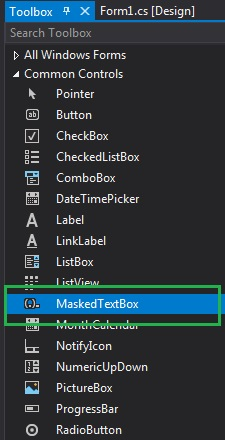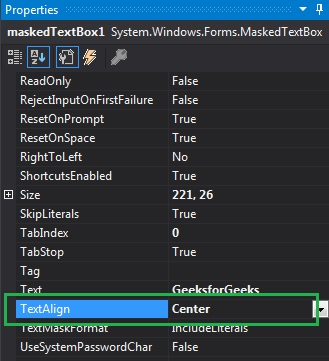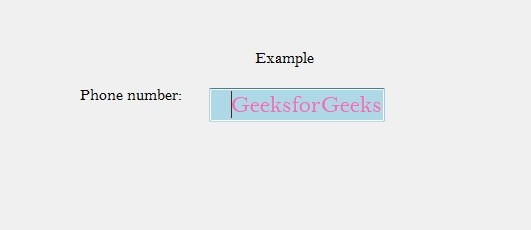Open In App

# How to set the Alignment of the Text in MaskedTextBox in C#?

In C#, MaskedTextBox control gives a validation procedure for the user input on the form like date, phone numbers, etc. Or in other words, it is used to provide a mask which differentiates between proper and improper user input. In MaskedTextBox control, you can set the alignment of the text in the MaskedTextBox using TextAlign Property which will be displayed to the user. This property has three values that are defined under HorizontalAlignment enum and the values are:

• Center value is used to align the text on the center of the control.
• Left value is used to align the text on the left side of the control.
• Right value is used to align the text on the right side of the control.

The default value of this property is Left. You can set this property in two different ways:

1. Design-Time: It is the easiest way to set the alignment of the text in the MaskedTextBox as shown in the following steps:

• Step 1: Create a windows form as shown in the below image:
Visual Studio -> File -> New -> Project -> WindowsFormApp• Step 2: Next, drag and drop the MaskedTextBox control from the toolbox on the form as shown in the below image:• Step 3: After drag and drop you will go to the properties of the MaskedTextBox and set the alignment of the text in the MaskedTextBox as shown in the below image:Output:2. Run-Time: It is a little bit trickier than the above method. In this method, you can set the alignment of the text in the MaskedTextBox control programmatically with the help of given syntax:

`public System.Windows.Forms.HorizontalAlignment TextAlign { get; set; }`

Here, HorizontalAlignment represents the values of this property. If the value of this property does not belong to HorizontalAlignment enum, then it will throw an InvalidEnumArgumentException. The following steps show how to set the alignment of the text in the MaskedTextBox dynamically:

```// Creating a MaskedTextBox
```
• Step 2: After creating MaskedTextBox, set the TextAlign property of the MaskedTextBox provided by the MaskedTextBox class.
```// Setting the text
m.TextAlign = HorizontalAlignment.Right;
```
• Step 3: And last add this MaskedTextBox control to the form using the following statement:
```// Adding MaskedTextBox
// control on the form
 `using` `System;``using` `System.Collections.Generic;``using` `System.ComponentModel;``using` `System.Data;``using` `System.Drawing;``using` `System.Linq;``using` `System.Text;``using` `System.Threading.Tasks;``using` `System.Windows.Forms;`` ` `namespace` `WindowsFormsApp38 {`` ` `public` `partial` `class` `Form1 : Form {`` ` `    ``public` `Form1()``    ``{``        ``InitializeComponent();``    ``}`` ` `    ``private` `void` `Form1_Load(``object` `sender, EventArgs e)``    ``{``        ``// Creating and setting the ``        ``// properties of the Label``        ``Label l1 = ``new` `Label();``        ``l1.Location = ``new` `Point(413, 98);``        ``l1.Size = ``new` `Size(176, 20);``        ``l1.Text = ``" Example"``;``        ``l1.Font = ``new` `Font(``"Bell MT"``, 12);`` ` `        ``// Adding label on the form``        ``this``.Controls.Add(l1);`` ` `        ``// Creating and setting the ``        ``// properties of the Label``        ``Label l2 = ``new` `Label();``        ``l2.Location = ``new` `Point(242, 135);``        ``l2.Size = ``new` `Size(126, 20);``        ``l2.Text = ``"Phone number:"``;``        ``l2.Font = ``new` `Font(``"Bell MT"``, 12);`` ` `        ``// Adding label on the form``        ``this``.Controls.Add(l2);`` ` `        ``// Creating and setting the ``        ``// properties of MaskedTextBox``        ``MaskedTextBox m = ``new` `MaskedTextBox();``        ``m.Location = ``new` `Point(374, 137);``        ``m.Text = ``"GeeksforGeeks"``;``        ``m.TextAlign = HorizontalAlignment.Right;``        ``m.Size = ``new` `Size(176, 20);``        ``m.Name = ``"MyBox"``;``        ``m.BorderStyle = BorderStyle.Fixed3D;``        ``m.BackColor = Color.LightBlue;``        ``m.ForeColor = Color.HotPink;``        ``m.Font = ``new` `Font(``"Bell MT"``, 18);`` ` `        ``// Adding MaskedTextBox``        ``// control on the form``        ``this``.Controls.Add(m);``    ``}``}``}`